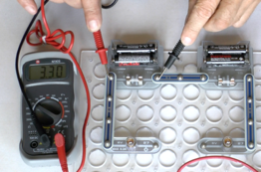Kirchoff’s Second Law

The potential difference (PD) or voltage measurement is all about the energy provided by the electricity.  The PD measures how much energy in joules is converted to heat or light by each coulomb of electricity passing.

If the current flowing is one amp(ere) then one coulomb per second is flowing through every part of the circuit. In this image the current is 0.31 amps so (fairly obviously) 0.31 coulombs is passing through the meter and every other part of the circuit each second. We have to measure the PD (voltage) to calculate the energy being provided each second.

The PD measures how much energy in joules is converted to heat or light by each coulomb of electricity passing, a PD of 1 volt shows that each coulomb is providing one joule of energy.

Here we are measuring the voltage across the cell when it is doing almost no work. Only a tiny current is flowing to operate the voltmeter but none is flowing around the bulbs because the circuit is incomplete. We are measuring the EMF which is a total of 6.6 volts for the four cells.But when we complete the circuit so that a current flows we are measuring the total potential difference (PD) around the components of the complete circuit where a current is flowing. The total is less than the 6.6 volts EMF

That is because the cells themselves have a resistance (the internal resistance) so some of the energy, that is some of the EMF, is used inside the cell. We cannot measure this value of voltage inside the cells directly but we know that it is 6.6 - 5.24 = 1.36 volts.